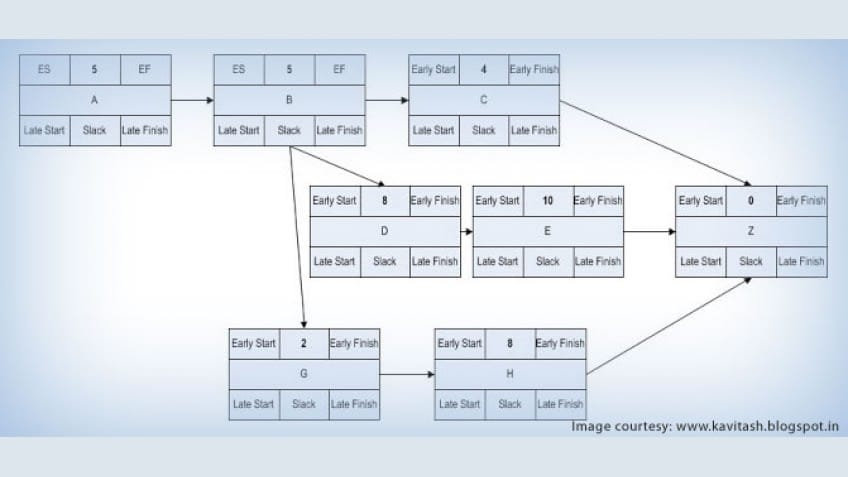Critical Path Method is a vital algorithm that is employed for scheduling a particular assemblage of project-related activities. A training program on PMP® Certification Training provides you with a better perspective on this technique. Learn about Critical Path Method in this video.

This technique is used to carry out the Schedule Network Analysis. This is again a very popular technique and we will study this method in next few slides, first, let me explain what a critical Path is? It is defined as the longest duration path through a network diagram and determines the shortest time to complete the project.

Float is another concept, which is quite important in understanding the critical path. Float is calculated once the network diagram is ready. There are three kind of float that can be calculated. The first is the Total Float, which denotes the amount of time an activity may be delayed without affecting the schedule of subsequent activities.

The second type of float is Free Float which is the amount of time an activity can be delayed without delaying the early start date of its successor(s) activities.

The last type is Project Float which is the amount of time a Project can be delayed without delaying the externally imposed project completion date required by the customer or previously committed by the Project Manager. You can think of float as a cushion time available to complete an activity. Float is also called slack. Now, let me ask you. What is the slack of the activities on the critical path? The answer is Zero.

There is no scope to delay activities in the critical path. Critical Path actually represents the Project duration. They actually add together to the Project duration, so where is the scope for the delay? Delaying activities on the critical path is as good as delaying the project duration!

There are two ways in which float of an activity can be calculated. But, before that, let me inform you that the first step in critical path method is to identify the Critical Path of the Network. Once the critical path is identified, calculate the start date and end date of each of the activities on the critical path.

As we have seen earlier, the float of the activities on the critical path is zero so they represent the overall project duration. Now that you know the Project duration, you can either go through the network starting with time zero and keep on calculating the time required to complete each activity till you reach the last activity of the Project. This is also called Forward pass. The starting time for each activity in this approach is called 'Early Start' and end time for each activity is called 'Early Finish'.

Or you can travel through the network from the project end date and calculate the time required to complete each activity. This is called backward pass. The end date in this approach is called the Late Finish and the start date of the activity in this approach is called the Late Start.

The float of the activity is either the difference between the 'Late Start' and 'Early Start' or the difference between the 'Late Finish' and 'Early Finish'. Both the differences work out to be the same. In case you find it difficult to understand critical path, I suggest that you go through the last two slides twice and then look at the next slide where we have explained a critical path example.

Insert Example Video

Let's look at this example. There are basically five activities in this Project. If you look at the Network diagram, there are two paths in this Network. Start, 1, 2, 4, 5, End is one path and Start, 1, 3, 5, End is the second path. Since the duration of the path Start, 1, 2, 4, 5, End is 18 days, which is more than the duration of the path Start, 1, 3, 5, End.

The critical path of the Project is Start, 1, 2, 4, 5, End. Take some time to calculate all the four values, i.e. Early Start, Early Finish, Late start and late finish of the activities. I have written down the values for your verification.

Let me take Activity 3 as an example.

First we will calculate the Early Start and Early Finish dates. Activity 3 can start only after Activity 1. Since the Early finish of Activity 1 is 3, which becomes the Early Start of Activity 3.Activity 3 cannot start earlier than 3, because Activity 1 is getting completed only then. So, Early Start of Activity 3 is 3. Since the duration of the activity is 4 days. The Early Finish of Activity 3 is 3+4= 7 days. Now, let's calculate the late start and late finish of Activity 3.

Late Start of Activity 5 is 14 days. The activity 3 happens just before Activity 5, so the Late Finish of Activity 3 is 14 days. To calculate the late start, you can subtract the duration from late finish. So the Late start of Activity 3 is 14-4=10.

We provide PMP® classroom training in the following cities:

 ALBUQUERQUE FAIRBANKS KANSAS CITY BATON ROUGE TORONTO PRAGUE ALBANY BEIJING MELBOURNE MANILA INDIANAPOLIS NASHVILLE EDISON KUWAIT TOKYO

PMP is a registered trademark of the Project Management Institute, Inc.Eshna